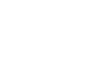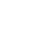How do we calculate capital gain tax? - letsdiskussAbdul Malik

News reporter (CEN News ) | Posted | Share-Market-Finance

# How do we calculate capital gain tax?

6
0Entrepreneur | Posted

The calculation process for short-term capital gain tax and long-term capital gain tax is slightly different.

Talking about the basics first, capital gains are gains arising from the sale of capital assets such as mutual funds, stocks, and real estate. Now there are two types of capital gains: short-term gains and long-term gains.

Short-term gains are gains coming from assets that you held for less than 36 months. Long-term capital gains are gains from assets that you held for more than 3 years. For equities and mutual funds, this period is 12 months though.

Short-term capital gain tax is easy to calculate actually. Just add your capital gain to your overall income and then estimate the tax according to the slab you fall in. As for the long-term capital gains, you also have to factor Cost Inflation Index, which is decided by the government every year.

Be Money Aware has a nice Capital Gain Calculator for FY 2017-18. Check it out here. Finotax, too, has a decent indexed cost and LTCG calculator. Check it here. Also, when you’re using such calculators to estimate your income tax, you would find easy options to input and calculate your capital gain tax.

So, point is, regardless how easy or difficult the calculation process is, avoid doing it manually to avoid errors and to be more efficient.4
0

Sales Manager... | Posted

Tax on long-term capital gain: Long-term capital gain is taxable at 20% + surcharge and education cess.

4
0

@letsuser | Posted

Calculate Capital Gains Formula
Short-term Capital Gains Tax:
In the case of short term capital gains, the computation is as given below:
Short-term capital gain= full value consideration – (cost of acquisition + cost of improvement + cost of transfer).

Long-term Capital Gains Tax:
To calculate the long-term capital gains tax payable, the following formula is to be used:
Long-term capital gain = full value of consideration received or accruing – (indexed cost of acquisition + indexed cost of improvement + cost of transfer), where:
Indexed cost of acquisition = cost of acquisition x cost inflation index of the year of transfer/cost inflation index of the year of acquisition.
Indexed cost of improvement = cost of improvement x cost inflation index of the year of transfer/cost inflation index of the year of improvement.

0
0

SEO(Search Engine Optiization) | Posted

Suppose You have the realized amount then, you have to minus the sales prize with any commissions paid. First, subtract your basic(what you have paid) from the realized amount ( how much you sold it for) then after determining the difference. If your assets is more than you paid then you have a capital gain.

0
0